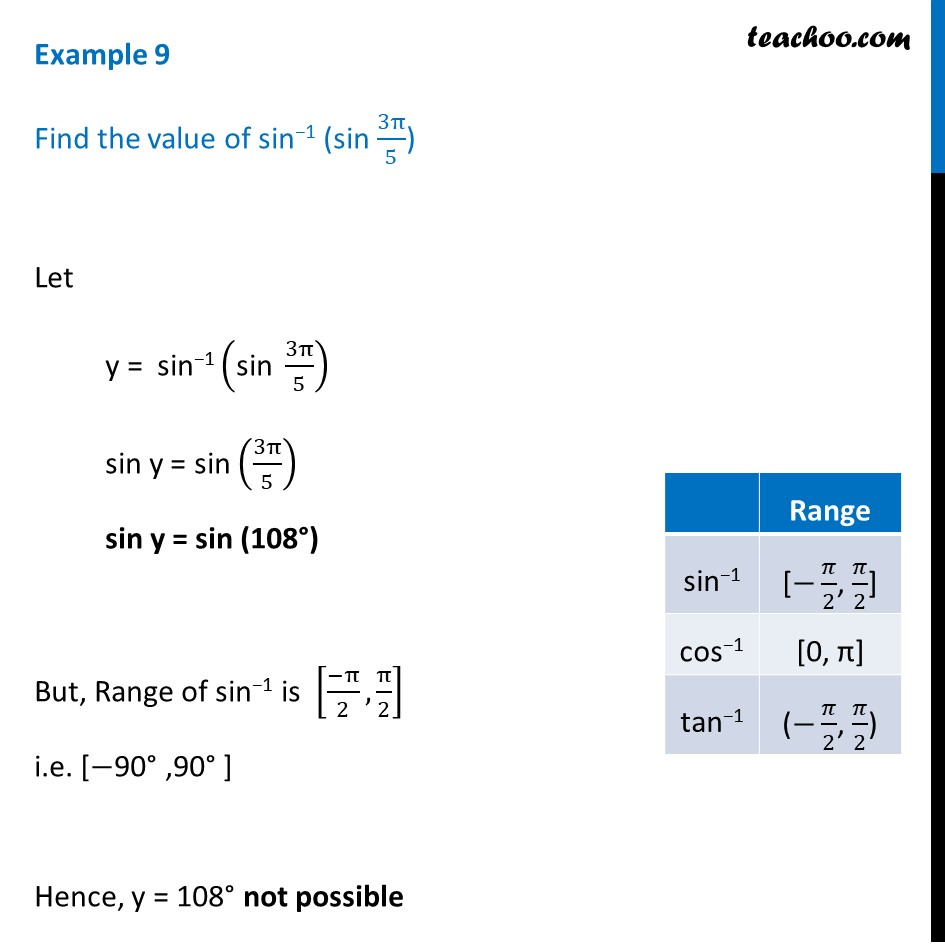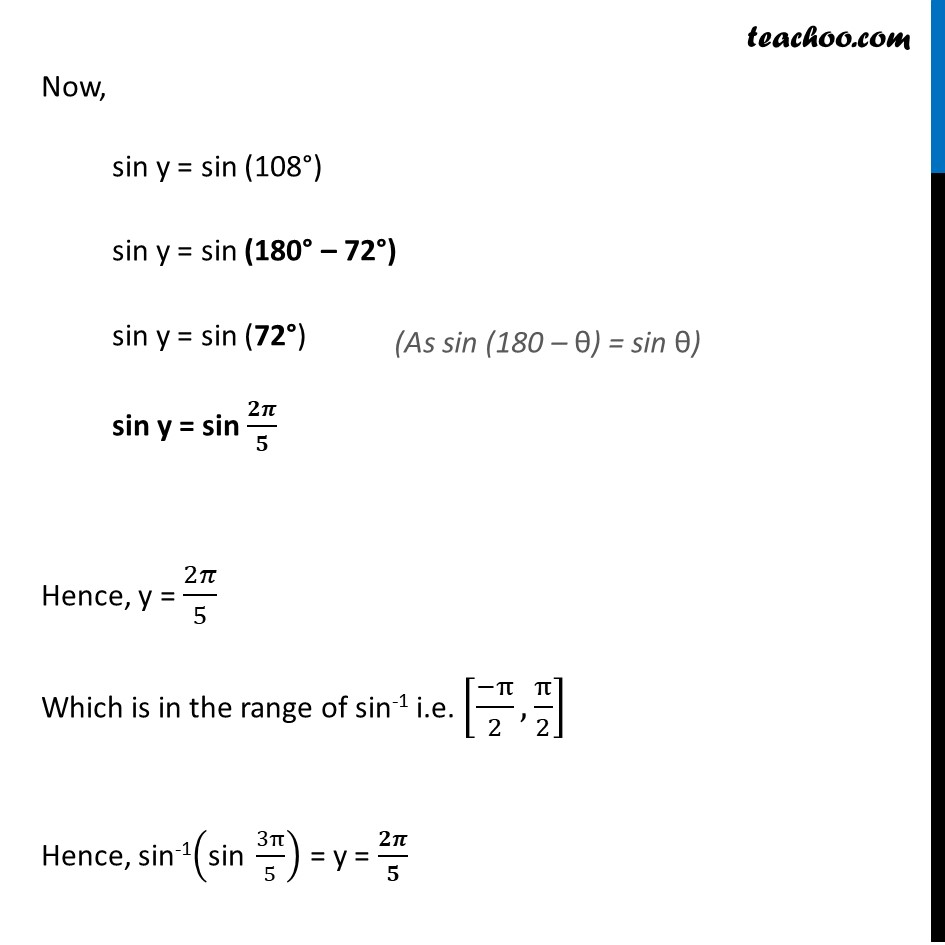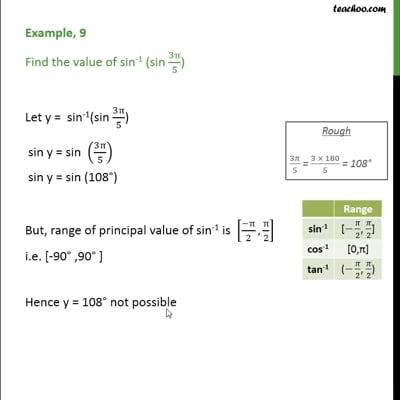Examples

Chapter 2 Class 12 Inverse Trigonometric Functions
Serial order wiseThis video is only available for Teachoo black users

Solve all your doubts with Teachoo Black (new monthly pack available now!)

### Transcript

Example 9 Find the value of sin−1 (sin 3π/5) Let y = sin−1 ("sin " 3π/5) sin y = sin (3π/5) sin y = sin (108°) But, Range of sin−1 is [(−π)/2, π/2] i.e. [−90° ,90° ] Hence, y = 108° not possible Now, sin y = sin (108°) sin y = sin (180° – 72°) sin y = sin (72°) sin y = sin 𝟐𝝅/𝟓 Hence, y = 2𝜋/5 Which is in the range of sin-1 i.e. [(−π)/2, π/2] Hence, sin-1("sin " 3π/5) = y = 𝟐𝝅/𝟓